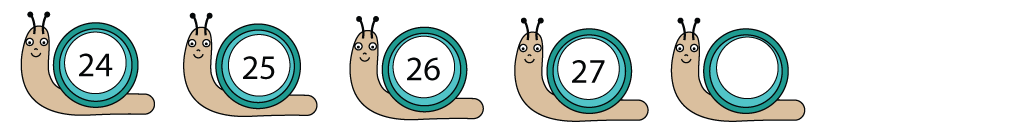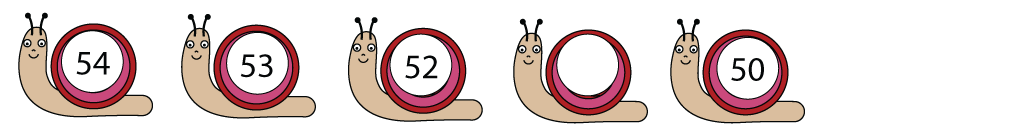A sequence is a pattern which is always the same.

Look at this example:The pattern here is to add 1 to each number.

27 + 1 is 28, so the next number is 28.

Look at this next example:The pattern here is to take 1 away from each number.

52 − 1 is 51, so the missing number is 51.

Now let's start the activities!